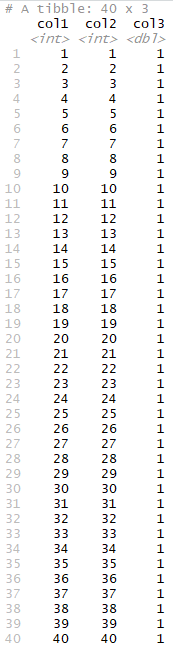Open in App
Not now

# Print Entire tibble to R Console

• Last Updated : 14 Sep, 2021

In this article, we are going to see how to print the entire tibble to R Console. A tibble is a well-organized data structure composed of rows and columns denoted by row names. Tibbles is displayed partially on the console displaying a small number of rows on the console.

## Method 1: Using dplyr library

The as_tibble() method in base R is used to access the data frame in the form of a tibble.

`as_tibble (data-frame)`

The nrow() method can be used to calculate the number of rows of the data frame or tibble.

`nrow(tibble)`

Using the print() method, the complete set of rows can be accessed using the second argument, which is equivalent to the number of rows of the tibble.

`print(tibble, nrow(tibble))`

Below is the implementation:

## R

 `library``(``"dplyr"``) ` ` `  `# creating a data frame ` `data_frame <- ``data.frame``(col1 = 1:40, ` `                       ``col2 = 1:40, ` `                       ``col3 = 1) ` ` `  `# converting to tibble ` `tib <- ``as_tibble``(data_frame) ` `print ``(``"Original Tibble"``) ` `print ``(tib) ` ` `  `# calculating number of rows of tibble ` `rows <- ``nrow``(tib) ` `print ``(``"Complete Tibble"``) ` `print``(tib,n=rows)`

Output:## Method 2: Using dplyr library and pipe operator

The %>% operator can be applied to the data frame using a sequence of operations. Initially, the as_tibble() method is applied on the data frame to convert to tibble and then printed using the n argument, set equivalent to the number of rows in the data frame.

Below is the implementation:

## R

 `library``(``"dplyr"``) ` ` `  `# creating a data frame ` `data_frame <- ``data.frame``(col1 = 1:40, ` `                       ``col2 = 1:40, ` `                       ``col3 = 1) ` `# converting to tibble ` `tib <- ``as_tibble``(data_frame) ` ` `  `print ``(``"Complete Tibble"``) ` `data_frame %>% ``as_tibble``() %>% ``print``(n=``nrow``(data_frame))`

Output:## Method 3: Using options() Method

The options() method in base R gives user the permission to set and examine a large number of global options. These options manipulate the way in which R computes and displays its results.

`options(...)`

The options can be used to set to any value, for instance using the dplyr package, it can be used to set to display a maximum length output.

`options(dplyr.print_max = 1e9)`

The modification of the global options is used to print the entire tibble on the console.

Below is the implementation:

## R

 `library``(``"dplyr"``) ` ` `  `# setting options ` `options``(dplyr.print_max = 1e9) ` ` `  `# creating a data frame ` `data_frame <- ``data.frame``(col1 = 1:40, ` `                       ``col2 = 1:40, ` `                       ``col3 = 1) ` ` `  `# converting to tibble ` `tib <- ``as_tibble``(data_frame) ` `print ``(``"Complete Tibble"``) ` `print``(tib)`

Output:My Personal Notes arrow_drop_up
Related Articles